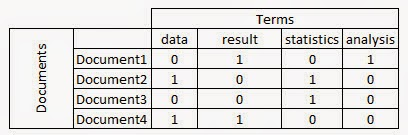# Text Mining Basics for Beginners

This tutorial covers basics and fundamentals of text mining. It includes detailed explanation of various text mining terms and terminologies. This tutorial is designed for beginners who are new to text analytics. It would help them to get started with text mining.

Text Mining Terminologies
1. Document is a sentence. For example, " Four score and seven years ago our fathers brought forth on this continent, a new nation, conceived in Liberty, and dedicated to the proposition that all men are created equal."
2. Tokens represent words. For example:  "nation", "Liberty", "men".
3. Terms may represent single words or multiword units, such as “civil war”
4. Corpus is a collection of documents (database). For example, A corpus contains 16 documents (16 txt files).
5. Stopwords are basically a set of commonly used words which you want to exclude while analyzing text. Examples of stopwords - 'a', 'an', 'the', 'to', 'of', 'ABC Company' etc.
6. Document Term Matrix is a matrix consisting of documents in a row and terms in columns
Example of document term matrix :Document Term Matrix

7. Sparse terms - Terms occurring only in very few documents (Sentences).

8. Tokenization - It is the process to divide unstructured data into tokens such as words, phrase, keywords etc.

9. Stemming -  For example, "interesting", "interest" and "interested" are all stemmed to "interest". After that, we can stem to their original forms, so that the words would look "normal".

10. Polarity - Whether a document or sentence is positive, negative or neutral. This term is commonly used in sentiment analysis.

11. Bag-of-words - Each sentence (or document) is a bag of words ignoring grammar and even word order. The terms ' make India' and 'India make' have the same probability score.

12. Part of Speech Tagging - It involves tagging every word in the document and assigns part of speech - noun, verb, adjective, pronoun, single noun, plural noun, etc.

13. Term Frequency - Inverse Document Frequency (tf-idf) -

It measures how important a word is.

It consists of two terms -
1. Term Frequency (tf)
2. Inverse Document Frequency (idf)
Term Frequency measures how frequently a word (term) occurs in a document.
TF(t) = (Number of times term t appears) / (Total number of terms).
Inverse Document Frequency measures how important a word is. If a word appears frequently in a document, then it should be important and we should give that word a high score. But if a word appears in too many other documents, it’s probably not a unique identifier, therefore we should assign a lower score to that word.
IDF(t) = log to base e(Total number of documents / Number of documents containing term t)
Term Frequency Inverse Document Frequency
tf-idf = tf × idf
Example : Suppose a word 'good' appears 373 times in total 6 documents which contains in total 122204 words (terms). Term Frequency (TF) would be 0.00305 i.e. =373/122204. But this word appears in only 1 document so IDF would be ln(6/1) = 1.791759. Hence, tf-idf = TF * IDF = 0.0054.

Uses of TF-IDF

1. Building Stopwords

Terms having tf-idf value zero or close to zero can be used in stop-words list. These are all words that appear in all of the documents, so the idf term is zero.

2. Important Words

Sort TF-IDF values in descending order. The term which appear at top after sorting is the most important word.

3. Text Clustering
• Calculate the tf-idf score for the collection of documents
• Calculate pairwise distance matrix using cosine distance algorithm
• Performs hierarchical clustering and visualize the clustering result with a dendrogram.

14. N-grams -

They are basically a set of co-occurring words within a given window.
• N-gram of size 1 - unigram
• N-gram of size 2 - bigram
• N-gram of size 3 - trigram
For Example, for the sentence "The cow jumps over the moon".
I. If N=2 (known as bigrams), then the n-grams would be:
the cow, cow jumps, jumps over, over the, the moon
In this case, we have 5 bigrams.

II. If N=3 (trigram), the n-grams would be:
the cow jumps, cow jumps over, jumps over the, over the moon

How many N-grams in a sentence?
If X=Number of words in a given sentence K, the number of n-grams for sentence K would be: N-grams = X – (N-1)
N-grams is used to use tokens such as bigrams in the feature space instead of just unigrams (one word). But various research papers warned the use of bigrams and trigrams in your feature space may not necessarily yield any significant improvement.
Trigrams vs. Bigrams
The Trigrams do have an advantage over bigrams but it is small.

Check out the detailed documentation : Trigrams and Bigrams ExplainedShare
Related PostsDeepanshu founded ListenData with a simple objective - Make analytics easy to understand and follow. He has over 10 years of experience in data science. During his tenure, he has worked with global clients in various domains like Banking, Insurance, Private Equity, Telecom and Human Resource.

5 Responses to "Text Mining Basics for Beginners"
1.Thanks for the Text Mining Basics. It helped to understand the topology of text analytics in R.

2.thank you for this quick overview of basic concepts!

3.Thank you, it really helped to get an idea on Text Mining Basics.

4.Thank you. Helped a lot to get the basics right

5.Next → ← Prev